ElMaestro
★★★

Denmark,
2010-02-06 17:10
(edited by ElMaestro on 2010-02-06 17:25)

Posting: # 4724
Views: 7,563

## Anticonservativism?! [Regulatives / Guidelines]

Bong sure,

referring to the other thread where the new BE guideline from EMEA is discussed and where HS proposed a simulation.

As usual I am several steps behind as I am not sure I understand all details of the discussion. Nevertheless, the word anticonservative was right in my face.
Can that claim be generalised in any way?

Following the philosophy of HS' single simulations experiment I think we could simulate different 3-per, 3-trt datasets under various conditions, analyse them by a mixed model and record sigma = the residual error (this is the s used in calculation of the conficence interval). Next, prune the dataset so it's possible to analyse it as a 2,2,2-BE dataset with a normal linear model, and then obtain the residual and compare. The philosophy here is that if the pruning results in a sigma that is on average larger than the sigma obtained through the full dataset, then the approach is conservative; otherwise it is not.
Here's some elmaestrolophystic code (probably bugged and wrong):

```load(nlme) nConservative=0 nAntiConservative=0 seq1 = as.factor(c(1,1,1, 2,2,2, 3,3,3, 4,4,4, 5,5,5, 6,6,6, 1,1,1, 2,2,2, 3,3,3, 4,4,4, 5,5,5, 6,6,6)) sub1 = as.factor(c(1,1,1, 2,2,2, 3,3,3, 4,4,4, 5,5,5, 6,6,6, 7,7,7, 8,8,8, 9,9,9, 10,10,10, 11,11,11, 12,12,12)) per1 = as.factor(c(1,2,3, 1,2,3, 1,2,3, 1,2,3, 1,2,3, 1,2,3, 1,2,3, 1,2,3,1,2,3, 1,2,3, 1,2,3, 1,2,3)) trt1 = as.factor(c(1,2,3, 2,3,1, 3,1,2, 1,3,2, 2,1,3, 3,2,1 ,1,2,3 ,2,3,1 ,3,1,2 ,1,3,2 ,2,1,3 ,3,2,1)) ##massacrating out everything related to R2 ##means that when T was before R1 we now have a seq 1 otherwise a seq 2 sub2 = as.factor(c(1,1,2,2,3,3,4,4,5,5,6,6,7,7,8,8,9,9,10,10,11,11,12,12)) per2 = as.factor(c(1,2,1,2,1,2,1,2,1,2,1,2,1,2,1,2,1,2,1,2,1,2,1,2)) trt2 = as.factor(c(1,2, 2,1 ,1,2, 1,2, 2,1, 2,1, 1,2, 2,1, 1,2, 1,2, 2,1, 2,1)) seq2 = as.factor(c(1,1, 2,2, 1,1, 1,1, 2,2, 2,2, 1,1, 2,2, 1,1, 1,1, 2,2, 2,2)) for (iter in 1:100) { ## T is 1, R1 is 2 and R2 is 3 lny1 = rnorm(n=36, mean=95, sd=0.15*95) for (i in 1:36) if (trt1[i]==2) lny1[i]=rnorm(n=1, mean = 90, sd = 0.10*90) for (i in 1:36) if (trt1[i]==3) lny1[i]=rnorm(n=1, mean = 110, sd = 0.15*110) ElMaestrosMixedModel= lme(lny1~0+seq1+per1+trt1, random = ~1|sub1) Smm=ElMaestrosMixedModel\$sigma ##do the pruning! lny2=lny1 length(lny2)=24 j=0 for (i in 1:36) if (trt1[i]!=3)   {     j=j+1;     lny2[j]=lny1[i]   } ElMaestrosLinearModel= lm(lny2~0+seq2+per2+trt2+sub2) Slm=summary(ElMaestrosLinearModel)\$sigma if (Slm<Smm) nAntiConservative=nAntiConservative+1 if (Slm>Smm) nConservative=nConservative+1 } cat("n AntiConservative = ", nAntiConservative, "\n") cat("n Conservative = ", nConservative, "\n")```

On my machine the code above is a win for anticonservativism. But that is dependent on the settings of means and CV in the treatment groups.
HS: I got the impression that you simulated your dataset on the ordinary scale, then log-transformed the data. At least, if I log-transform your data then I can reproduce your result (with the code above). Here I am simulating normal dist data on the log scale. Seems more right to me.

Bugs galore....

EM.

Update a few mins after posting: Seems escape characters do not go well on this forum?! The last two lines starting with "cat" was intended to finish with a letter n preceded by a down-right slash but I cannot paste that for some reason.

Edit: Yeah, that's a bug (see here). Corrected in the v.1.7.7beta scripts - hopefully available here soon. [HS]
Helmut
★★★Vienna, Austria,
2011-11-03 21:12

@ ElMaestro
Posting: # 7598
Views: 6,129

## Conservativism?

Hi to all simulants!

I modified your code according to Martin's suggestion. I tested three scenarios (10 000 sims each):
• CVT=CVR1=CVR2=30% (conventional setup)
• CVT=CVR2=30%, CVR1=50%
• CVT=CVR1=30%, CVR2=50%
In all simulations T/R1=95%, T/R2=100/0.95≈105.27%. I’m not sure whether the setup of CVs is correct – seems to be CVtotal.

```require(nlme) set.seed(12041958) # keep this line to compare results only sims   <- 10000    # <- no of sims here # T/R1 = 95%, T/R2 = 105.26% mean1 <- 1.00;   cv1 <- mean1/100*30 # <- means & CVs here mean2 <- 0.95;   cv2 <- mean2/100*30 # <- mean3 <- 1/0.95; cv3 <- mean3/100*30 # <- seq1 <- as.factor(rep(rep(1:6, each = 3), 2)) sub1 <- as.factor(rep(1:12, each = 3)) per1 <- as.factor(rep(1:3, 12)) trt1 <- as.factor(rep(c(1,2,3, 2,3,1, 3,1,2, 1,3,2, 2,1,3, 3,2,1), 2)) # massacrating out everything related to R2 # means that when T was before R1 we now have a seq 1 otherwise a seq 2 sub2 <- as.factor(rep(1:12, each = 2)) per2 <- as.factor(rep(1:2, 12)) trt2 <- as.factor(rep(c(1,2, 2,1, 1,2, 1,2, 2,1, 2,1), 2)) seq2 <- as.factor(rep(c(1,1, 2,2, 1,1, 1,1, 2,2, 2,2), 2)) # see Martin's post: http://forum.bebac.at/mix_entry.php?id=5272 meanl1 <- log(mean1)-0.5*log(cv1^2+1) meanl2 <- log(mean2)-0.5*log(cv2^2+1) meanl3 <- log(mean3)-0.5*log(cv3^2+1) sdl1   <- sqrt(log(cv1^2+1)) sdl2   <- sqrt(log(cv2^2+1)) sdl3   <- sqrt(log(cv3^2+1)) nConservative <- 0; nAntiConservative <- 0 for (iter in 1:sims) # T is 1, R1 is 2 and R2 is 3   {   y1 = rlnorm(n=36, meanlog=meanl1, sdlog=sdl1)   for (i in 1:36) if (trt1[i]==2) y1[i]=rlnorm(n=1, meanlog=meanl2, sdlog=sdl2)   for (i in 1:36) if (trt1[i]==3) y1[i]=rlnorm(n=1, meanlog=meanl3, sdlog=sdl3)   ElMaestrosMixedModel <- lme(y1 ~ 0 + seq1 + per1 + trt1, random = ~1|sub1)   Smm <-ElMaestrosMixedModel\$sigma   # do the pruning!   y2 <- y1   length(y2) <- 24   j <- 0   for (i in 1:36) if (trt1[i] != 3)     { j     <- j+1;       y2[j] <- y1[i]     }   HSLinearModel <- lm(y2 ~ 0 + seq2 + sub2 %in% seq2 + per2 + trt2) # EMA?!   Slm <- summary(HSLinearModel)\$sigma   if (Slm<Smm) nAntiConservative <- nAntiConservative + 1   if (Slm>=Smm) nConservative    <- nConservative + 1   } cat(" Conservative = ", 100*nConservative/sims, "%\n","Anticonservative = ", 100*nAntiConservative/sims, "%\n Based on",sims,"simulated BE studies; n=12 each.","\n")```

Is this correct – or did I bungle the code?```        T  R1  R2   Cons.   Anticons. Sc.1  30  30  30   44.05%   55.95% Sc.2  30  50  30   63.42%   36.58% Sc.3  30  30  50   14.11%   85.89%```

Cheers,
Helmut SchützThe quality of responses received is directly proportional to the quality of the question asked. ☼
Science Quotes
ElMaestro
★★★

Denmark,
2011-11-03 21:43

@ Helmut
Posting: # 7599
Views: 6,048

## In hindsight...

Hi HS,

2 comments.

1:
» `ElMaestrosMixedModel <- lme(y1 ~ 0 + seq1 + per1 + trt1, random = ~1|sub1)`
I am not sure why ElMaestro used a mixed model here; doesn't this specification just give the same as a linear model with seq1, per1, trt1, and sub1 as fixed factors?

2:
I think we need to take degrees of freedom into consideration too. After all, the critical value of the t-dist (and thus the width of the CI which is the ultimate indicator) depends heavily on it. I think the df's differ between the two scenarios. I hope this does not lead into a discussion about how df's are calculated for a mixed model (R will not do Satterthwaite etc.), a discussion where I have absolutely nothing to contribute.

I am sorry to question my own sanity.

``` if (3) 4 x=c("Foo", "Bar") b=data.frame(x) typeof(b[,1]) ##aha, integer? b[,1]+1 ##then let me add 1```

Best regards,
ElMaestro

“(...) targeted cancer therapies will benefit fewer than 2 percent of the cancer patients they’re aimed at. That reality is often lost on consumers, who are being fed a steady diet of winning anecdotes about miracle cures.” New York Times (ed.), June 9, 2018.
Helmut
★★★Vienna, Austria,
2011-11-04 01:56

@ ElMaestro
Posting: # 7601
Views: 6,268

## In hindsight...

Salute!

» I am not sure why ElMaestro used a mixed model here; doesn't this specification just give the same as a linear model with seq1, per1, trt1, and sub1 as fixed factors?

In terms of the residual variance – yes. Good ol’ days of subjects as random. Met last week another statistical pro who was upset upon the ‘all fixed’ story.» I think we need to take degrees of freedom into consideration too. […] I think the df's differ between the two scenarios.

Right.

» I hope this does not lead into a discussion about how df's are calculated for a mixed model (R will not do Satterthwaite etc.),

No, no. Everything is balanced here. 20 dfs in the Williams’ design and 10 after R2 is thrown out.

Another try:

```set.seed(12041958) # keep this line to compare results only sims   <- 10000    # <- no of sims here # T/R1 = 95%, T/R2 = 105.26% mean1 <- 1.00;   cv1 <- mean1/100*30 # <- means & (total!) CVs here mean2 <- 0.95;   cv2 <- mean2/100*30 # <- mean3 <- 1/0.95; cv3 <- mean3/100*30 # <- seq1 <- as.factor(rep(rep(1:6, each = 3), 2)) sub1 <- as.factor(rep(1:12, each = 3)) per1 <- as.factor(rep(1:3, 12)) trt1 <- as.factor(rep(c(1,2,3, 2,3,1, 3,1,2, 1,3,2, 2,1,3, 3,2,1), 2)) # massacrating out everything related to R2 # means that when T was before R1 we now have a seq 1 otherwise a seq 2 sub2 <- as.factor(rep(1:12, each = 2)) per2 <- as.factor(rep(1:2, 12)) trt2 <- as.factor(rep(c(1,2, 2,1, 1,2, 1,2, 2,1, 2,1), 2)) seq2 <- as.factor(rep(c(1,1, 2,2, 1,1, 1,1, 2,2, 2,2), 2)) # see Martin's post: http://forum.bebac.at/mix_entry.php?id=5272 meanl1 <- log(mean1)-0.5*log(cv1^2+1); sdl1 <- sqrt(log(cv1^2+1)) meanl2 <- log(mean2)-0.5*log(cv2^2+1); sdl2 <- sqrt(log(cv2^2+1)) meanl3 <- log(mean3)-0.5*log(cv3^2+1); sdl3 <- sqrt(log(cv3^2+1)) Liberal <- 0; Conserv <- 0 FMCVintra <- NULL; RMCVintra <- NULL for (iter in 1:sims) # T is 1, R1 is 2 and R2 is 3   {   y1 = rlnorm(n=36, meanlog=meanl1, sdlog=sdl1)   for (i in 1:36) if (trt1[i]==2) y1[i]=rlnorm(n=1,meanlog=meanl2,sdlog=sdl2)   for (i in 1:36) if (trt1[i]==3) y1[i]=rlnorm(n=1,meanlog=meanl3,sdlog=sdl3)   FullModel <- lm(y1 ~ 0 + seq1 + sub1 %in% seq1 + per1 + trt1)   FMDelta   <- mean(y1[trt1==1])-mean(y1[trt1==2])   FMdf      <- aov(FullModel)\$df.residual   FMMSE     <- summary(FullModel)\$sigma^2/FMdf   FMlo      <- 100*exp(FMDelta - qt(1-0.05,FMdf)*sqrt(2*FMMSE/12))   FMhi      <- 100*exp(FMDelta + qt(1-0.05,FMdf)*sqrt(2*FMMSE/12))   FMCI      <- FMhi - FMlo   FMCVintra <- c(FMCVintra, sqrt(exp(FMMSE)-1))   # do the pruning!   y2 <- y1   length(y2) <- 24   j <- 0   for (i in 1:36) if (trt1[i] != 3)     { j     <- j+1;       y2[j] <- y1[i]     }   ReducedModel <- lm(y2 ~ 0 + seq2 + sub2 %in% seq2 + per2 + trt2) # EMA?!   RMDelta   <- mean(y2[trt2==1])-mean(y2[trt2==2])   RMdf      <- aov(ReducedModel)\$df.residual   RMMSE     <- summary(ReducedModel)\$sigma^2/RMdf   RMlo      <- 100*exp(RMDelta - qt(1-0.05,RMdf)*sqrt(2*RMMSE/12))   RMhi      <- 100*exp(RMDelta + qt(1-0.05,RMdf)*sqrt(2*RMMSE/12))   RMCI      <- RMhi - RMlo   RMCVintra <- c(RMCVintra, sqrt(exp(RMMSE)-1))   if (RMCI  < FMCI){Liberal <- Liberal + 1}else{Conserv <- Conserv + 1} } result     <- paste(paste(" Eliminated R2 (EMA) compared to full model (Williams’ design)\n"),               paste("Conservative: ", 100*Conserv/sims,               "%, CVintra: ",round(100*median(FMCVintra), digits=2),"%\n"),               paste("Liberal:      ", 100*Liberal/sims,               "%, CVintra: ",round(100*median(RMCVintra), digits=2),"%\n"),               paste("Based on",sims,"simulated BE studies; n=12 each.","\n")) cat(result)```

Sorry for the lengthy code; couldn’t do better.

```       T  R1  R2   Cons. (CVintra)  Liberal (CVintra) Sc.1  30  30  30   91.57% (6.56%)   8.43% ( 8.58%) Sc.2  30  50  30   96.90% (7.83%)   3.10% (11.27%) Sc.3  30  30  50   62.87% (8.26%)  37.13% ( 8.58%) ```
Hhm. Kannitverstan.

Cheers,
Helmut SchützThe quality of responses received is directly proportional to the quality of the question asked. ☼
Science Quotes
ElMaestro
★★★

Denmark,
2011-11-04 08:41

@ Helmut
Posting: # 7602
Views: 5,989

## In hindsight...

Good morning HS,

I might add:

At initialisation:
``` RM.be <- 0 FM.be <- 0```

At evaluation:
```if ((FMlo>=80) && (FMhi<=125)) FM.be <- FM.be + 1 if ((RMlo>=80) && (RMhi<=125)) RM.be <- RM.be + 1```

...and then compare FM.be and RM.be at exit.

And now it's time for the second cup of coffee. Have a great day.

EM.
d_labes
★★★

Berlin, Germany,
2011-11-04 09:40

@ Helmut
Posting: # 7603
Views: 6,029

## rlnorm simulates what?

Dear Helmut!

One fundamental question:
If you simulate with rlnorm you get log-normal distributed values. Like those for AUC and Cmax in BE studies were also a log-normal distribution is assumed.

The logs of the values simulated with rlnorm will be normally distributed.
So long so good. Nothing new told here.

But now how to analyse them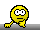?
Your code f.i. the line
» ` FullModel <- lm(y1 ~ 0 + seq1 + sub1 %in% seq1 + per1 + trt1)`
or what I would do
` FullModel <- lm(log(y1) ~ 0 + seq1 + sub1 %in% seq1 + per1 + trt1)`

Regards,

Detlew
Helmut
★★★Vienna, Austria,
2011-11-04 16:05

@ d_labes
Posting: # 7607
Views: 6,158

## Oops.

Dear simulants!

» or what I would do
» ` FullModel <- lm(log(y1) ~ 0 + seq1 + sub1 %in% seq1 + per1 + trt1)`

Oh THX! Affects also the T-R. Latest version (flexible sample sizes, corrected T/R1 and T/R2):

```set.seed(12041958) # keep this line to compare results only sims   <- 10000    # <- no of sims size   <- 12       # <- no of subjects # T/R1 = 95%, T/R2 = 105.26% mean1 <- 0.95;   cv1 <- mean1/100*30 # <- means & (total!) CVs here mean2 <- 1.00;   cv2 <- mean2/100*30 # <- mean3 <- 0.95^2; cv3 <- mean3/100*30 # <- seq1 <- as.factor(rep(rep(1:6, each = 3), size/6)) sub1 <- as.factor(rep(1:size, each = 3)) per1 <- as.factor(rep(1:3, size)) trt1 <- as.factor(rep(c(1,2,3, 2,3,1, 3,1,2, 1,3,2, 2,1,3, 3,2,1), size/6)) # massacrating out everything related to R2 # means that when T was before R1 we now have a seq 1 otherwise a seq 2 seq2 <- as.factor(rep(c(1,1, 2,2, 1,1, 1,1, 2,2, 2,2), size/6)) sub2 <- as.factor(rep(1:size, each = 2)) per2 <- as.factor(rep(1:2, size)) trt2 <- as.factor(rep(c(1,2, 2,1, 1,2, 1,2, 2,1, 2,1), size/6)) # see Martin's post: http://forum.bebac.at/mix_entry.php?id=5272 meanl1 <- log(mean1)-0.5*log(cv1^2+1); sdl1 <- sqrt(log(cv1^2+1)) meanl2 <- log(mean2)-0.5*log(cv2^2+1); sdl2 <- sqrt(log(cv2^2+1)) meanl3 <- log(mean3)-0.5*log(cv3^2+1); sdl3 <- sqrt(log(cv3^2+1)) RM.be  <- 0; FM.be <- 0 FMCVintra <- NULL; RMCVintra <- NULL for (iter in 1:sims) # T is 1, R1 is 2 and R2 is 3   {   y1 = rlnorm(n=size*3, meanlog=meanl1, sdlog=sdl1)   for (i in 1:size*3) if (trt1[i ]==2) y1[i ]=rlnorm(n=1,meanlog=meanl2,sdlog=sdl2)   for (i in 1:size*3) if (trt1[i ]==3) y1[i ]=rlnorm(n=1,meanlog=meanl3,sdlog=sdl3)   FullModel <- lm(log(y1) ~ 0 + seq1 + sub1 %in% seq1 + per1 + trt1)   FMDelta   <- mean(log(y1)[trt1==1])-mean(log(y1)[trt1==2])   FMdf      <- aov(FullModel)\$df.residual   FMMSE     <- summary(FullModel)\$sigma^2/FMdf   FMlo      <- 100*exp(FMDelta - qt(1-0.05,FMdf)*sqrt(2*FMMSE/size))   FMhi      <- 100*exp(FMDelta + qt(1-0.05,FMdf)*sqrt(2*FMMSE/size))   FMCI      <- FMhi - FMlo   FMCVintra <- c(FMCVintra, sqrt(exp(FMMSE)-1))   if ((FMlo>=80) && (FMhi<=125)) FM.be <- FM.be + 1   # do the pruning!   y2 <- y1   length(y2) <- size*2   j <- 0   for (i in 1:size*3) if (trt1[i ] != 3)     { j     <- j+1;       y2[j] <- y1[i ]     }   ReducedModel <- lm(log(y2) ~ 0 + seq2 + sub2 %in% seq2 + per2 + trt2) # EMA?!   RMDelta   <- mean(log(y2)[trt2==1])-mean(log(y2)[trt2==2])   RMdf      <- aov(ReducedModel)\$df.residual   RMMSE     <- summary(ReducedModel)\$sigma^2/RMdf   RMlo      <- 100*exp(RMDelta - qt(1-0.05,RMdf)*sqrt(2*RMMSE/size))   RMhi      <- 100*exp(RMDelta + qt(1-0.05,RMdf)*sqrt(2*RMMSE/size))   RMCI      <- RMhi - RMlo   RMCVintra <- c(RMCVintra, sqrt(exp(RMMSE)-1))   if ((RMlo>=80) && (RMhi<=125)) RM.be <- RM.be + 1 } if (RM.be>FM.be){Outcome <- "liberal."}else{Outcome <- "conservative."} result <- paste(paste(           " Reduced model (EMA: R2 dropped) compared to full model (Williams’ design)\n"),           paste("Full model passed BE:    ",           format(100*FM.be/sims,digits=4,nsmall=2,zero.print=TRUE),           "%, CVintra: ",           format(100*median(FMCVintra),digits=2,nsmall=2,           zero.print=TRUE),"%\n"),           paste("Reduced model passed BE: ",           format(100*RM.be/sims,digits=4,nsmall=2,zero.print=TRUE),           "%, CVintra: ",           format(100*median(RMCVintra),digits=2,nsmall=2,           zero.print=TRUE),"%\n"),           paste("Assessment: Reduced model is",Outcome,"\n"),           paste("Based on",sims,"simulated BE studies; n =",size,"each.","\n")) cat(result)```

Results:
```       T  R1  R2   Full BE (CVintra)  Red. BE (CVintra) Sc.1  30  30  30   87.40% (6.17%)    82.22% ( 8.69%) Red. = conservative Sc.2  30  50  30   81.63% (6.71%)    70.28% (10.31%) Red. = conservative Sc.3  30  30  50   86.61% (6.65%)    78.43% ( 9.19%) Red. = conservative```

I have no idea how to simulate CVintra; CVtotal is not what I really want (to see if reference’s different variances have an influence on the result).

Cheers,
Helmut SchützThe quality of responses received is directly proportional to the quality of the question asked. ☼
Science Quotes
ElMaestro
★★★

Denmark,
2011-11-04 16:22
(edited by ElMaestro on 2011-11-04 22:36)

@ Helmut
Posting: # 7610
Views: 6,041

## Oops.

Hi Hs,

» I have no idea how to simulate CVintra; CVtotal is not what I really want (to see if reference’s different variances have an influence on the result).

Neither had I when I started this thread, but now I have and I don't think it is difficult. Our model (simplest case but not satisfying d_labes' concern):

y~Seq+Subj+Treat+Per+error

There is one error in play unless we do a mixed model. So we sample the error (intrasubject) from a normal distribution and add it to the fixed effects. We can set the fixed effects to zero for Per and Seq, and tweak the Treat's as per our wishes for T/R ratios.

Edit:
The following code possibly shows what I suggest (and I am as always probably wrong):
``` Per=c(1,2,3, 1,2,3, 1,2,3, 1,2,3, 1,2,3, 1,2,3, 1,2,3, 1,2,3, 1,2,3, 1,2,3, 1,2,3, 1,2,3) Subj=c(1,1,1, 2,2,2, 3,3,3, 4,4,4, 5,5,5, 6,6,6, 7,7,7, 8,8,8, 9,9,9, 10,10,10, 11,11,11, 12,12,12) Trt=c(1,2,3,1,2,3,1,3,2,1,3,2,2,1,3,2,1,3,2,3,1,2,3,1,3,2,1,3,2,1,3,1,2,3,1,2) Seq=c("ABC","ABC","ABC","ABC","ABC","ABC","ACB","ACB","ACB","ACB","ACB", "ACB","BAC","BAC","BAC","BAC","BAC","BAC","BCA","BCA","BCA","BCA","BCA", "BCA","CBA","CBA","CBA","CBA","CBA","CBA","CAB","CAB","CAB","CAB","CAB","CAB") intraCV =0.3 ##30 percent CV ##CVintra=sqrt(exp(MSE)-1) MSE=log(1+intraCV^2) Trt.effects=c(0.9, 1.0, 0.95) ## or whatever the truly should be cf. Martin's suggestion ##a simulation loop should start here! ## now we make the y vector AUC=rnorm(36,  mean=0, sd=sqrt(MSE)) ##first the error/noise for (i in 1:36)  {    foo=Trt[i]    AUC[i]= AUC[i]+Trt.effects[foo] ##and here we add the fixed effect  }```

##..and analyse from here

I have not debugged so it is prolly full of errors.

``` if (3) 4 x=c("Foo", "Bar") b=data.frame(x) typeof(b[,1]) ##aha, integer? b[,1]+1 ##then let me add 1```

Best regards,
ElMaestro

“(...) targeted cancer therapies will benefit fewer than 2 percent of the cancer patients they’re aimed at. That reality is often lost on consumers, who are being fed a steady diet of winning anecdotes about miracle cures.” New York Times (ed.), June 9, 2018.
d_labes
★★★

Berlin, Germany,
2011-11-07 11:16

@ ElMaestro
Posting: # 7631
Views: 6,109

## Simulation of intra-subject variability

Dear Ol' Pirate, dear Helmut,

» » I have no idea how to simulate CVintra; CVtotal is not what I really want (to see if reference’s different variances have an influence on the result).
»
» Neither had I when I started this thread, but now I have and I don't think it is difficult. Our model (simplest case but not satisfying d_labes' concern):
»
» y~Seq+Subj+Treat+Per+error
»
» There is one error in play unless we do a mixed model. So we sample the error (intrasubject) from a normal distribution and add it to the fixed effects. We can set the fixed effects to zero for Per and Seq, and tweak the Treat's as per our wishes for T/R ratios.

Totally right if you think in terms of the EMA mantra 'all effects fixed'. But then you have to specify subjects effects in some reasonable way. What you do is to assume subjects effect of zero or Dolly-clones of a subjecthaving all the same subject effect.

Don't know how this affects your goal.

Don't also know what a reasonable way is to specify subjects fixed effects. At least you have many, many scenarios beside those you like to consider.

The way out would be to consider subjects effects as random with a CV associated. Of course this is a mixed model. No one else than EMA has doubt about it.
Refer f.i. to Chow, Liu "Design and Analysis of Bioavailability ..." page 42 for the model of the classical 2x2 crossover.

To generalize this to the 3x3 study one could simulate the logs of a PK metric via a multivariate normal distribution with variance-covariance matrix
```  ( varT+varS  varS        varS       )  ( varS       varR1+varS  varS       )  ( varS       varS        varR2+varS ) ```
where varT, varR1 and varR2 are the intra-subject variances and varS the variability of the subjects effect (between-subjects variability).
This model of course neglects any subject-by-treatment interaction.
Together with a proper specification of the mean vector (µT, µR1, µR2) you get from the multivariate normal distribution (R package mvtnorm) vectors of simulated logs for T, R1 and R2.
In this notation one neglects also period effects. If you like to deal with them you have to write down the above variance-covariance matrix for the period values for each sequence analogous to Chow, Liu for the 2x2 crossover.
Another possibility would be the simulation with a zero mean vector and add the necessary fixed effects (treatment, period) afterwards.

Hope this gibberish sounds reasonable to you and I have not made a big mistake.
Code follows if I have tested it.

Regards,

Detlew
ElMaestro
★★★

Denmark,
2011-11-08 11:02
(edited by ElMaestro on 2011-11-08 17:16)

@ d_labes
Posting: # 7634
Views: 5,900

## Simulation of intra-subject variability

All hands on deck!

I hope this illustrates the issue of no treament differences when fixed effects between subjects are zerod or some scatter in fixed effects between subjects exists:
```Per=c(1,2,3, 1,2,3, 1,2,3, 1,2,3, 1,2,3, 1,2,3, 1,2,3, 1,2,3, 1,2,3, 1,2,3, 1,2,3, 1,2,3) Subj=c(1,1,1, 2,2,2, 3,3,3, 4,4,4, 5,5,5, 6,6,6, 7,7,7, 8,8,8, 9,9,9, 10,10,10, 11,11,11, 12,12,12) Trt=c(1,2,3,1,2,3,1,3,2,1,3,2,2,1,3,2,1,3,2,3,1,2,3,1,3,2,1,3,2,1,3,1,2,3,1,2) Seq=c("ABC","ABC","ABC","ABC","ABC","ABC","ACB","ACB","ACB","ACB","ACB", "ACB","BAC","BAC","BAC","BAC","BAC","BAC","BCA","BCA","BCA","BCA","BCA", "BCA","CBA","CBA","CBA","CBA","CBA","CBA","CAB","CAB","CAB","CAB","CAB","CAB") Seq=sort(rep(1:6, 6)) SubjE1=c(rep(0,12)) ##fixed effects for subjs: zero for all 12 SubjE2=c(9*runif(12)) ##variation in fixed effects between subjects TrtE=c(9.876, 8.765, 7.654) ## some fixed treatment effects PerE=c(1.5, 1.5, 1.5) ##some fixed period effects all same SeqE=c(2.0,2.0, 2.0, 2.0, 2.0, 2.0) ##some fixed sequence effects all same set.seed(12041958) rany = rnorm(36, mean=0, sd=2) ##our single error Y1=c(1:36) Y2=c(1:36) for (i in 1:36)  {   indexTrt=Trt[i];   indexSubj=Subj[i];   indexPer=Per[i];   indexSeq=Seq[i];   rany =  rnorm(1, mean=0, sd=2)   Y1[i] = TrtE[indexTrt] + SeqE[indexSeq] + SubjE1[indexSubj] + PerE[indexPer]+rany   Y2[i] = TrtE[indexTrt] + SeqE[indexSeq] + SubjE2[indexSubj] + PerE[indexPer]+rany  } m1=lm(Y1~0+as.factor(Trt) + as.factor(Seq)+ as.factor(Subj) + as.factor(Per)) m2=lm(Y2~0+as.factor(Trt) + as.factor(Seq)+ as.factor(Subj) + as.factor(Per)) anova(m1) cat("m1 no subject effect. They do not differ in this scenario.\n") anova(m2) cat("m2 p significant for subjects. They differ in this scenario.\n") cat("m1 models all subjects fixed effects as zero.\n") cat("m1 difference between treat effects 1 and 3:", coef(m1)-coef(m1), "\n") cat("m1 residual error:", summary(m1)\$sigma, "\n") cat("m3 models subjects effects as fixed but different between subjects.\n") cat("m2 difference between treat effects 1 and 3:", coef(m2)-coef(m2), "\n") cat("m2 residual error:", summary(m2)\$sigma, "\n") # #```

Have a good day. As always it is prolly wrong and bugged.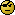EM

``` if (3) 4 x=c("Foo", "Bar") b=data.frame(x) typeof(b[,1]) ##aha, integer? b[,1]+1 ##then let me add 1```

Best regards,
ElMaestro

“(...) targeted cancer therapies will benefit fewer than 2 percent of the cancer patients they’re aimed at. That reality is often lost on consumers, who are being fed a steady diet of winning anecdotes about miracle cures.” New York Times (ed.), June 9, 2018.
d_labes
★★★

Berlin, Germany,
2011-11-25 13:54

@ Helmut
Posting: # 7723
Views: 5,648

## Oops. Oops.

Dear Helmut!

Quite a time ago I had promised to come out with a simulation including subject variability and intra-subject correlation.

Due to spare time I could not until now consider this.
Before posting my results I would like to compare it to your code.

Inspecting it I have some doubts:
» `# massacrating out everything related to R2`
» `# means that when T was before R1 we now have a seq 1 otherwise a seq 2`
» `seq2 <- as.factor(rep(c(1,1, 2,2, 1,1, 1,1, 2,2, 2,2), size/6))`
» `sub2 <- as.factor(rep(1:size, each = 2))`
» `per2 <- as.factor(rep(1:2, size))`
» `trt2 <- as.factor(rep(c(1,2, 2,1, 1,2, 1,2, 2,1, 2,1), size/6))`

Must the EMA guidance interpreted in this way, i.e. analysing the dataset with 'pseudo' periods and sequences? Or should it interpreted the way which is employed in the Q&A for the replicate design (intra-subject variability of the reference). That means only massacre out the data concerning R2 but retaining the original periods and sequences.» `FullModel <- lm(log(y1) ~ 0 + seq1 + sub1 %in% seq1 + per1 + trt1)`
» `FMDelta   <- mean(log(y1)[trt1==1])-mean(log(y1)[trt1==2])`
» `FMdf      <- aov(FullModel)\$df.residual`
» `FMMSE     <- summary(FullModel)\$sigma^2/FMdf`
» `FMlo      <- 100*exp(FMDelta - qt(1-0.05,FMdf)*sqrt(2*FMMSE/size))`
» `FMhi      <- 100*exp(FMDelta + qt(1-0.05,FMdf)*sqrt(2*FMMSE/size))`
» `FMCI      <- FMhi - FMlo`
» `FMCVintra <- c(FMCVintra, sqrt(exp(FMMSE)-1))`

At least the code for FMMSE has stolen my sleep. According to the help page of `lm.summary()` "... sigma - the square root of the estimated variance of the random error" and IMHO then the MSE is sigma^2 without the division by the degrees of freedom!
Check this out via `anova(FullModel)["Residuals","Mean Sq"]==summary(FullModel)\$sigma^2 `.
Or did I miss sumfink here?

Regards,

Detlew
Helmut
★★★Vienna, Austria,
2011-11-25 14:23

@ d_labes
Posting: # 7724
Views: 5,666

## Another Oops.

Dear Detlew!

» Inspecting it I have some doubts:
» » […] some nonsense code
»
» Must the EMA guidance interpreted in this way, i.e. analysing the dataset with 'pseudo' periods and sequences? Or should it interpreted the way which is employed in the Q&A for the replicate design (intra-subject variability of the reference). That means only massacre out the data concerning R2 but retaining the original periods and sequences.Exactly! Should forget copypasting old code when new ‘knowledge’ became available in the meantime.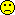» » `FMMSE     <- summary(FullModel)\$sigma^2/FMdf`
» At least the code for FMMSE has stolen my sleep. According to the help page of `lm.summary()` "... sigma - the square root of the estimated variance of the random error" and IMHO then the MSE is sigma^2 without the division by the degrees of freedom!

Oh s**t!

» Check this out via `anova(FullModel)["Residuals","Mean Sq"]==summary(FullModel)\$sigma^2 `.
» Or did I miss sumfink here?

No, no. Sorry!

Cheers,
Helmut SchützThe quality of responses received is directly proportional to the quality of the question asked. ☼
Science Quotes
d_labes
★★★

Berlin, Germany,
2011-11-04 15:46

@ ElMaestro
Posting: # 7606
Views: 6,007

## Simul Ants questions

Dear EM,

» `load(nlme)````  nConservative=0  nAntiConservative=0    ...  for (iter in 1:100)  {    ## T is 1, R1 is 2 and R2 is 3  lny1 = rnorm(n=36, mean=95, sd=0.15*95)  for (i in 1:36) if (trt1[i]==2) lny1[i]=rnorm(n=1, mean = 90, sd = 0.10*90)  for (i in 1:36) if (trt1[i]==3) lny1[i]=rnorm(n=1, mean = 110, sd = 0.15*110)  ... ```

Really?
I would suggest:
If you consider 95, 90 and 110 as the arithmetic means on the untransformed scale use log(x)-sd2/2 as the mean of the logs.
If your numbers 0.15, 0.10 and 0.15 are the CV's user sd = sqrt(log(CV*CV+1)) as sd of the logs.

The manner you have written the simulation for the three treatments suggest you consider the log(metric) for the 3 treatments as independent.
Is this really the case for a cross-over where the 3 treatment were applied to each subject? IMHO we have to consider some correlation between them.

Regards,

Detlew
ElMaestro
★★★

Denmark,
2011-11-04 16:06

@ d_labes
Posting: # 7608
Views: 6,003

## Simul Ants questions

Hi d_labes,

» Really?
» I would suggest:
» If you consider 95, 90 and 110 as the arithmetic means on the untransformed scale use log(x)-sd2/2 as the mean of the logs.
» If your numbers 0.15, 0.10 and 0.15 are the CV's user sd = sqrt(log(CV*CV+1)) as sd of the logs.

I think Martin raised this before. I don't understand this aspect. Feel free to educate me, you great scientist!

» The manner you have written the simulation for the three treatments suggest you consider the log(metric) for the 3 treatments as independent.
» Is this really the case for a cross-over where the 3 treatment were applied to each subject? IMHO we have to consider some correlation between them.

Probably a good point. But let's take the simple 2,2,2-BE scenario as example. Treatmeant T and R is probably correlated within patients; but we still only work with a covariance matrix with a single sigma squared on the diagonal and zeros elsewhere. I don't know how to take any such correlation into effect, but if you wish to do it a way forward might be a mixed model.

As you can see above the original code was something I wrote 20 months ago, and I am today questioning my sanity back then. Actually I am still bonkers, but I guess that's another matter.

EM.
Helmut
★★★Vienna, Austria,
2011-11-04 16:09

@ d_labes
Posting: # 7609
Views: 6,024

## Simul Ants questions

Dear Detlew!

» The manner you have written the simulation for the three treatments suggest you consider the log(metric) for the 3 treatments as independent.
» Is this really the case for a cross-over where the 3 treatment were applied to each subject? IMHO we have to consider some correlation between them.

Exactly. Saw your post after submitting mine. See its end…Cheers,
Helmut SchützThe quality of responses received is directly proportional to the quality of the question asked. ☼
Science Quotes
d_labes
★★★

Berlin, Germany,
2011-11-08 11:31

@ ElMaestro
Posting: # 7635
Views: 5,968

## Liberal Conservatives

Moin moin Simul-Ants!

Sitting here, being bored, wondering about the meaning of liberal (anti-conservative), conservative within the context discussed here. Sure we don't do dirty politics here.

I have found this online resource and this one on the topic.
I think this has a direct influence on the scenarios to simulate here, namely we have to simulate ratios on the acceptance borders (H0: inequivalence) to get an estimate of the type I error alpha.

Otherwise we simulate some sort of power at the set ratios (near 1) I think. And higher power may be an indication of a liberal test but must not. It can also be that the alpha is <5% (conservative) but the test has more power.

BTW: post 499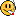.

Regards,

Detlew
martin
★★

Austria,
2011-11-08 20:50

@ d_labes
Posting: # 7640
Views: 5,838

## Liberal Conservatives

Dear d_labes!

You may find the following paper of interest where the terms "liberal" and "conservative" are used for evaluation of the simulation results.

Jaki T, Wolfsegger MJ, Lawo J-P (2010). Establishing bioequivalence in complete and incomplete data designs using AUCs. Journal of Biopharmaceutical Statistics, 20(4):803–820. DOI: 10.1080/10543401003618835.

A pre-print of this article can be found here.

hope this helps

Martin
Helmut
★★★Vienna, Austria,
2011-11-08 22:56

@ martin
Posting: # 7641
Views: 5,901

## Liberal Conservatives

Dear Martin!

THX for reminding me on this paper – I remember your presentation at the Inst. for Biostat. also quite well.I overlooked this sentence in the discussion:

The total sample size in the complete data design is smaller than for the serial sampling design, with the advantage disappearing with increasing intra-subject correlation with little effect of the number of measured time points to estimate the AUC. (my emphasis)

I looked into my database and evaluated the within-subject correlation in 130+ studies and close to 3,000 subjects. I considered only studies showing BE (I can hear you shout: “Selection bias!!”). Not surprisingly correlations were quite high. Forgot to bring the data to Berlin. I will post some results ASAP.Cheers,
Helmut SchützThe quality of responses received is directly proportional to the quality of the question asked. ☼
Science Quotes
martin
★★

Austria,
2011-11-09 09:01

@ Helmut
Posting: # 7642
Views: 5,831

## intra-subject correlation

Dear HS!

This is extremely interesting. I would by happy if you can you provide some summary regarding the correlation between time points. (e.g. was the correlation constant over time, ...).

best regards

Martin

PS.: As far as I know, Thomas will give a presentation on flexible batch designs at the Inst. for Biostat in the near future. I hope that you will find some time to participate as your input is highly appreciated!! I suppose that there will be also a debriefing at the old AKH.Helmut
★★★Vienna, Austria,
2011-11-25 17:16

@ martin
Posting: # 7725
Views: 5,832

## intra-subject correlation

Dear Martin!

» This is extremely interesting. I would by happy if you can you provide some summary regarding the correlation between time points. (e.g. was the correlation constant over time, …).

As I told you last Wednesday at the ‘debriefing’ I would need a month of spare time to retrieve them (~100,000 data pairs in various formats). I can only serve with an overview. 133 cross-over studies, 2843 subjects, AUCt or AUCτ:```              min      2.5%     med      97.5%    max R²            0.0480   0.1905   0.6517   0.9111   0.9639 R²adj         0.0047   0.1606   0.6329   0.9022   0.9623 sample size   6       12       20       48       52```

R² of my sample has an interesting distribution…

Of course, correlation depends on the sample size* – but you get an impression.
Studies with low correlations contained one ‘outlier’ each but were still large enough to show BE (well, that was my selection bias).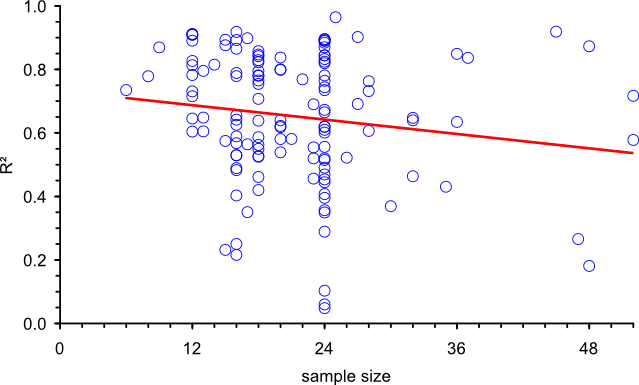Sponsors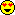n=24.

• Odeh RE. Critical values of the sample product-moment correlation coefficient in the bivariate distribution. Commun Statist–Simula Computa.192;11(1):1–26.

Cheers,
Helmut SchützThe quality of responses received is directly proportional to the quality of the question asked. ☼
Science Quotes
Bioequivalence and Bioavailability Forum |  Admin contact
19,488 posts in 4,135 threads, 1,336 registered users;
online 6 (0 registered, 6 guests [including 4 identified bots]).
Forum time (Europe/Vienna): 01:09 CEST

No rational argument will have a rational effect on a man
who does not want to adopt a rational attitude.    Karl R. Popper

The BIOEQUIVALENCE / BIOAVAILABILITY FORUM is hosted byIng. Helmut Schütz